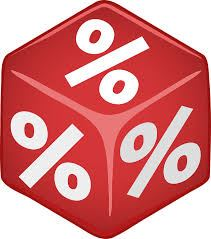# Ratio To Percentage

Ratio to percentage is the conversion procedure, which helps to convert the ratio into a percentage. As we know, the ratio is used to compare two quantities of the same unit. Whereas, a percentage is a specific type of ratio in which the value of the whole is always equal to 100. In this article, let us discuss how to convert ratio to percentage, formula, and the ratio to percentage value for some of the most frequently used numbers with many solved examples in detail.

## Ratio to Percentage Conversion

Ratio to percentage conversion helps us in obtaining accuracy in mixtures of elements, or while calculating the percentage score in a test. At times you are given parts of a quantity in the form of ratios. They can also be represented in the form of percentages. Let us understand this with the help of examples.Ratio to Percentage Conversion

Go through the following procedure to understand how to convert the ratio to percentage:

Step 1: First write the ratio a:b in the form of fraction a/b.

Step 2: Multiply the fraction a/b by 100 to convert in terms of percentage.

Step 3: Finally, add the percentage symbol (%) to the resultant value.

For example, to convert the ratio 5: 4, follow the below steps:

Step 1: The ratio 5:4 is written as 5/4.

Step 2: Multiply 5/4 by 100. (i.e) (5/4)×100 = 125.

Step 3: Adding the percentage symbol (%) to the resultant value, we get 125%.

The ratio 5:4 is written as 125%.

## Ratio to Percentage Formula

The ratio to percentage formula is used to convert the ratio to percentage. The ratio to percentage formula is given as follows:

Percentage = Ratio × 100

The percentage is represented using the symbol %.

### Ratio to Percentage Table

The ratio to percentage table provides the percentage value for some of the most commonly used ratios. Go through the following ratio to percentage values.

 Ratio Percentage Conversion Percentage 1: 2 (1/2) ×100 50% 1: 3 (1/3) ×100 33.33% 1: 4 (1/4) ×100 25% 1: 5 (1/5) ×100 20% 1: 10 (1/10) ×100 10% 2: 5 (2/5) ×100 40% 4: 5 (4/5) ×100 80% 1: 50 (1/50) ×100 2% 1: 100 (1/100) ×100 1%

### Ratio to Percent Examples

Example 1:

Convert the ratio 2:1 into percentage.

Solution:

Given ratio: 2:1

Step 1: The ratio 2:1 is written as 2/1.

Step 2: Multiplying 2/1 by 100 we get 200. (i.e) (2/1)×100 = 200.

Step 3: Adding the percentage symbol to the resultant value, we get the answer as 200%.

Therefore, 2:1 = 200%.

Example 2:

Varun received his monthly salary. The ratio of his expenditure to savings is 7:3. What percentage of his salary, did he spend and what percentage was saved by him?

Solution:

Since the part of saving and expenditure are 3 and 7, the salary can be taken as 3 + 7 = 10 parts. This implies, 7/10 part of the salary is spent whereas 3/10 parts are saved.

Converting ratio to percentage we get,

Percentage of expenditure = 7/10 x 100% = 70%

Similarly, percentage of savings = 3/10 x 100% = 30%

Try This: Ratio To Percent Calculator

Example 3:

The angles of a triangle are in the ratio 1:1:2. Find the value of each angle. What will be the percentage of each angle?

Solution:

Since the angles are in ratio 1:1:2, there are 1 + 1 + 2 = 4 parts. The sum of angles in a triangle is 180 degrees.

Thus, the measure of the first angle = 1/4 x 180 = 45 degrees

Measure of the second angle = 1/4 x 180 = 45 degrees

Measure of the third angle = 2/4 x 180 = 90 degrees

Similarly, converting ratio to percentage we have,

First angle = 1/4 x 100% = 25%

Second angle = 1/4 x 100% = 25%

Third angle = 2/4 x 100% = 50%

## Frequently Asked Questions on Ratio to Percentage

Q1

### What is the ratio to percentage formula?

The ratio to percentage formula is:
Percentage = Ratio ×100

Q2

### Mention the steps to convert ratio to percentage?

The steps to convert the ratio to percentage are:
Write the given ratio in fractional form.
Multiply the fraction by 100
Simplify the obtained expression and add a percentage symbol to the resultant value.

Q3

### Convert the ratio 4:1 to percentage.

The percentage value of 4:1 is calculated as follows:
4:1 = (4/1) ×100 = 400%
Thus, the percentage of the ratio 4:1 is 400%

Q4

### Convert 75% into ratio.

75% = 75/100 = 3/4
Therefore, 75% = 3: 4.

Q5

### What is 25% as a ratio?

25% = 25/100 = ¼.
Therefore, the ratio equivalent to 25% is ¼.

Test your Knowledge on Ratio To Percentage